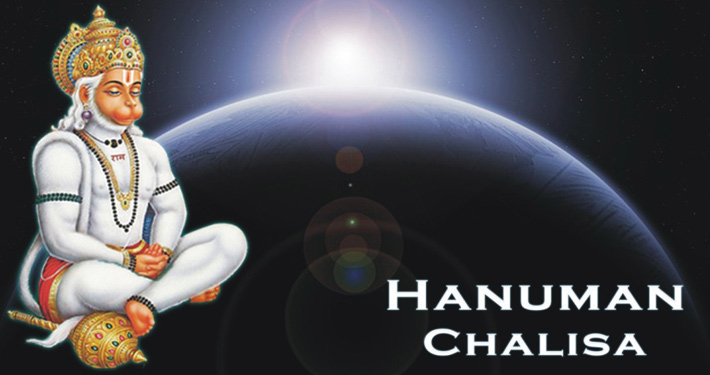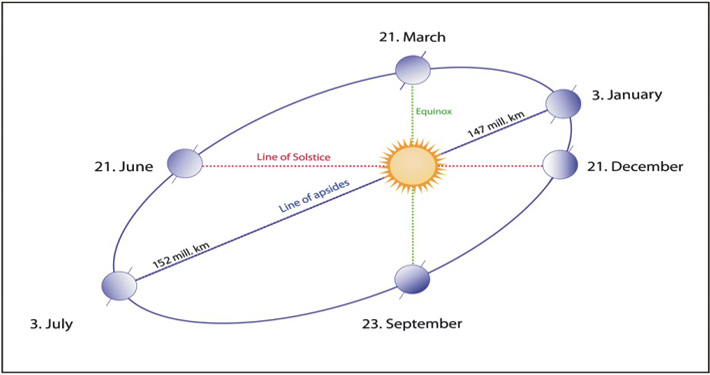Search

# Hanuman Chalisa predicts the distance between Sun and Earth

Do you know that the distance between the Sun and Earth is mentioned in Hanuman Chalisa with the accuracy of Vedic calculations?Hanuman Chalisa was composed by Gosvami Tulasidasa. He was a great devotee of Lord Ramachandra who lived in the 16th century. He composed the Rama-Charita-Manasa, the epic story of Lord Rama retold in the vernacular language. Many devotees regularly recite Hanuman Chalisa, a prayer glorifying Shri Hanuman, composed by this great saint and poet.

It is believed that in one of these verses of Hanuman Chalisa, Tulasidasa had given an accurate calculation of the distance between the Sun and Earth.

#### The Quest of Astronomers to find the distance of Sun

The Greek Astronomers were known for their contribution to the scientific field in understanding the heavenly bodies.

• Archimedes, an ancient Greek Mathematician, and Philosopher of the 3rd century BC, estimated the distance of Sun from Earth as 10000 times the radius of Earth.
• Later, Hipparchus (2nd century BC) gave an estimate of 490 times the radius of Earth.
• Ptolemy considered the distance to be 1210 times the radius of Earth.

However, Johannes Kepler (1571 – 1630), a German Mathematician and Astronomer realized that these estimates were significantly low. Kepler’s law of Planetary Motion allowed astronomers to calculate the relative distance of the planets from Sun. This was also aided by the invention of the telescope in the beginning of the 17th century which helped them to get more accurate measurements.

However, the most modern calculations in 20th century estimate the distance to be somewhere around 23455 times the radius of Earth (149,431,805 km assuming the radius of Earth to be 6371 km).

Srila Prabhupada writes in one of his purports:
Modern scientific calculations are subject to one change after another, and therefore they are uncertain. We have to accept the calculations of the Vedic literature. These Vedic calculations are steady; the astronomical calculations made long ago and recorded in the Vedic literature are correct even now. Whether the Vedic calculations or modern ones are better may remain a mystery for others, but as far as we are concerned, we accept the Vedic calculations to be correct.

#### According to the modern calculation:The average distance between the Sun and Earth =149 million km = 92 million miles.

However, the orbit of the Earth is not a perfect circle, but an ellipse. Sometimes the Earth is closer to the Sun and sometimes it is farther.The shortest distance between Sun and Earth (perihelion) = 91 million miles = 147 million km (early January)

The longest distance between Sun and Earth (aphelion) = 94.5 million miles = 152 million km (early July)

It is surprising to note that Tulasidasa who lived in the 16th century could give the most accurate estimation that is very close to the estimation by 20th-century astronomers.

Let us decipher the calculation in Hanuman Chalisa…

Hanuman, in his childhood, assuming the Sun to be a ripe mango, jumped to catch it. Tulasidasa recounts this incident in his Hanuman Chalisa as follows:

yuga-sahasra-yojana para bhanu

Considering the Sun to be a sweet fruit, Hanuman jumped to swallow it.

Here the distance he traveled is mentioned as yuga-sahasra-yojana. Let us try to decipher this.

What is a yuga? According to Bhagavad-gita, one day of Brahma is called Kalpa and is equal to 1000 yugas and this is followed by a similar duration of the night.

ratrim yuga-sahasrantamte ‘ho-ratra-vidojanah

1 yuga = 4,320,000 years = 12000 divine years

(1 divine year = 360 years according to human calculation)

This is also confirmed in Manu-samhita:

According to the above verse from Hanuman Chalisa, the distance between Sun and Earth is:

yuga-sahasra-yojana = 12000 x 1000 yojanas.

Yojana is a Vedic measure of distance and approximately equals to 8 miles (according to the 14th-century scholar Parameshvara, the originator of drgganita system). And 1 mile = 1.60934 kilometers.

#### According to the calculation presented in Hanuman Chalisa

Distance between Sun and Earth = 12000 x 1000 yojanas = 96 million miles = 153.6 million km, which is much closer to the calculation of the modern scientists.

A question may arise here.

Yuga is a measure of time and Yojana is a measure of distance. How can these two be combined?

We see that even modern scientists use time measurement (light-years) to calculate distances that are too long. It is said that the light from the sun takes approximately 8 minutes to reach the Earth.

The velocity of the light is calculated as 3 x 108 mps. In 8 minutes, the light travels (3 x 108 x 60 x 8) meters = 1.44 x 1011 meters = 1.44 x 108 km = 144 million kilometers (which is another approximation of distance between sun and earth according to modern calculations).

The assumptions we have made in the above calculations are as follows:

• We assumed yuga to mean the number 12000 based on the time calculation system of Vedic period based on the statement from Bhagavad-gita and Manu Samhita.
• We approximated 1 yojana = 8 miles based on what Srila Prabhupada has mentioned in his purports. However, there is still disagreement among scholars as to whether it is 5 miles or 8 miles. Some other calculations indicate values ranging from 7.6 miles to 8.5 miles.

But it is astonishing that Tulasidasa mentioned the distance to this level of accuracy as early as in 16th century when the Western astronomers, with the help of a telescope, were trying to figure out the distance.